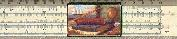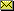# Negative Times Negative is What?

### Copyright (c) 1997 by Kenny Felder

Mike, a ninth grader in Westbrook, Maine, emailed me the question: "Why is it when you multiply two negative numbers you get a positive number?" I thought that was a great question, because it shows that Mike really wanted to understand the rules, not just use them!

I actually have four very different answers to the same question. The first is cute and easy to follow, but it may leave you unconvinced. The second is my favorite: not hard to follow, very convincing, and (best of all) very concrete. The third one demonstrates a good way of dealing with negative numbers in general, which is to start with positive numbers and work down. And the fourth is for people who like to "prove" things.

### The First Answer: Grammatical Analogy

Some people think of negative as meaning "not." So if I say "I am not going to the store," that is sort of the negative version of "I am going to the store."

So what do two "nots" mean? Consider this sentence: "You may tell me NOT to go to the store, but I'm NOT going to do what you say!" By negating your negation, I am insisting that I WILL go to the store.

OK, you get the idea? Two "nots" cancel each other out, just like two negatives. That was the appetizer: here comes the main course...

### The Second Answer: Money Analogy

One way to think of numbers is in terms of money. Let's say you and I are playing poker. To make life convenient, we use chips instead of real money. A green chip is worth \$5. A red chip means that you owe \$5. So if you lose \$5, you can represent that by giving up a green chip, or (if you're out) by picking up a red chip. Of course, you are always allowed to pick up a green chip and a red chip at the same time, because that doesn't change your total sum. (At the end, presumably, we'll cash in all our chips and see who gains or loses what money.)

I hope you see the mathematical analogy I'm drawing here: A green chip represents +\$5, and a red chip represents -\$5. Make sense? If so, here comes multiplication in terms of chips.

If you gain three green chips, what happens? Intuitively, you know that you gain \$15. Mathematically, we take the +\$5 that one chip is worth, and multiply it by +3, to indicate that you are gaining three chips. 3 x \$5 = \$15; positive times positive is positive.

If you gain three red chips, what happens? If you think about it, I think you'll agree that you just lost \$15. Mathematically, this looks the same as the previous example, except that the +\$5 that represents a green chip, is replaced by -\$5 to represent a red chip. 3 x -\$5 = -\$15; positive times negative is negative.

Now, what if you lose three green chips? Once again, you have lost \$15. We represent this "loss" mathematically by changing the 3 into a -3, so our equation is: -3 x \$5 = -\$15. Negative times positive is negative.

And finally, what if you lose three red chips? Hooray! This is happy news, it means you have actually gained money. Mathematically, this is -3 (since you lost) times -\$5 (since they were red chips). -3 x -\$5 = +\$15. Negative times negative is positive.

### The Third Answer: Progress from the Positive Numbers

Another way to think about negative numbers is that they "continue the sequence" as positive numbers go down. In other words, if I go from 4 to 3 to 2 to 1 to 0 and then keep going, I get into the negative numbers. Nothing should change drastically when I make that switch.

So, consider what happens when I take all the numbers in that sequence, and multiply them by 5:

4 x 5 = 20
3 x 5 = 15
2 x 5 = 10
1 x 5 = 5
0 x 5 = 0

What's happening on the left is that the numbers are going down by one. What's happening on the right is that the numbers are going down by 5.

If we keep dropping the left-hand column by one, we expect the trend to continue. Hence, the next few terms are...

-1 x 5 = -5
-2 x 5 = -10
-3 x 5 = -15

...and so on. So this tells us that a negative times a positive is a negative.

Now, let's do the same thing with the multiples of -5 instead of 5.

4 x -5 = -20
3 x -5 = -15
2 x -5 = -10
1 x -5 = -5
0 x -5 = 0

Once again, on the left, our terms are going down by 1. But what's happening on the right? The terms are going up by 5 each time. (If you don't believe this, think of them as temperatures. When you go from -20 to -15, the temperature has gone up by 5.) So if that sequence continues, we will see...

-1 x -5 = 5
-2 x -5 = 10
-3 x -5 = 15

Once again, a negative times a negative is a positive.

This method doesn't have the punchy mnemonic quality of the first answer, or the rigor of the fourth. And it doesn't convey powerful intuition as well as the second answer, which is my favorite. But it does have this benefit: if you start to think about negative numbers this way, as what happens to positive numbers when you count down, you can also answer other questions such as "What do negative exponents mean?" (In other words, what is 5-3?)

### The Fourth Answer: A Proof of Sorts

Finally, for people who like algebraic sorts of proofs, consider this. A and B are both positive numbers. I will start with a statement that is definitely true, and then I will multiply both sides by B.

A + (-A) = 0
(A)(B) + (-A)(B) = 0

Since the term on the left is positive, the term on the right must be negative. (I'm assuming here that if two non-zero numbers add up to zero, then one of them is negative and one is positive—I think that is too obvious to require a proof.) This proves that negative times positive is negative. Now:

A + (-A) = 0
(A)(-B) + (-A)(-B) = 0

Since the term on the left is negative (as we just proved!), the term on the right must be positive.

For another cool page on this same topic, see www.mathsisfun.com/multiplying-negatives.htmlGary and Kenny Felder's Math and Physics Help Home Page
`www.felderbooks.com/papers`Send comments or questions to the author